# 树---二叉树(创建，求高，大小，遍历)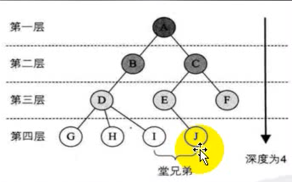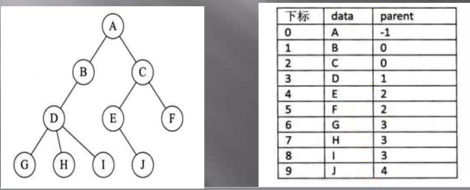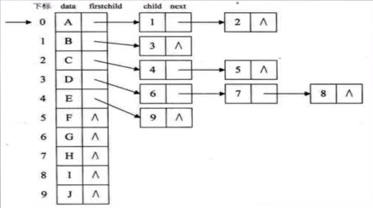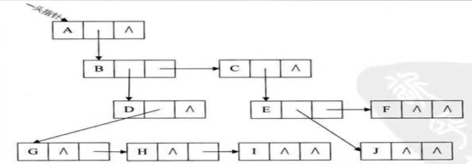class TreeNode {
private int index;
private String value;
//左右孩子初始值默认为null，因为建立这个点时，我们不知道其孩子的信息
private TreeNode leftChild = null;
private TreeNode rightChild = null;
}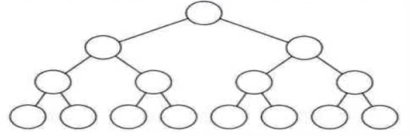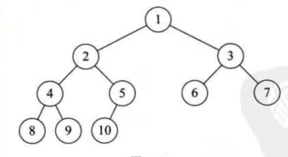（1）如果i = 1，则结点i是二叉树的根，无双亲；如果i > 1,则其双亲是结点[i / 2]

（2）如果i = 1, 则结点i无左孩子(结点i为叶子结点)，否则其左孩子是结点2i

（3）如果2i + 1 > n，则结点无右孩子，否则其右孩子结点为2i + 1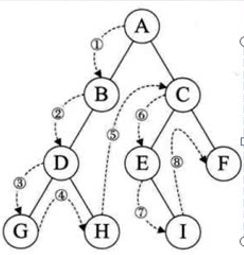public static void preNode(TreeNode node) {
if(node == null) {
return;
}
System.out.println(node.getValue());
preNode(node.leftChild);
preNode(node.rightChild);
}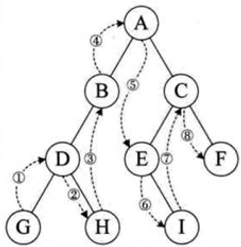public static void midNode(TreeNode node) {
if(node == null) {
return;
}

midNode(node.leftChild);
System.out.println(node.getValue());
midNode(node.rightChild);
}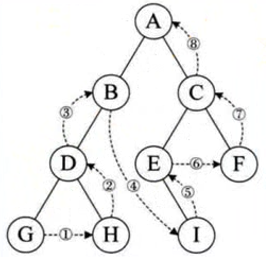public static void subNode(TreeNode node) {
if(node == null) {
return;
}

subNode(node.leftChild);
subNode(node.rightChild);
System.out.println(node.getValue());
}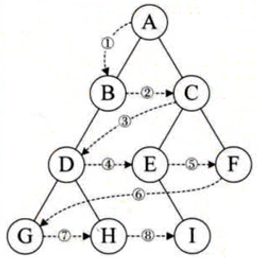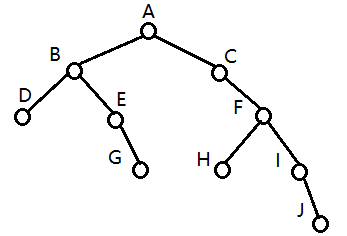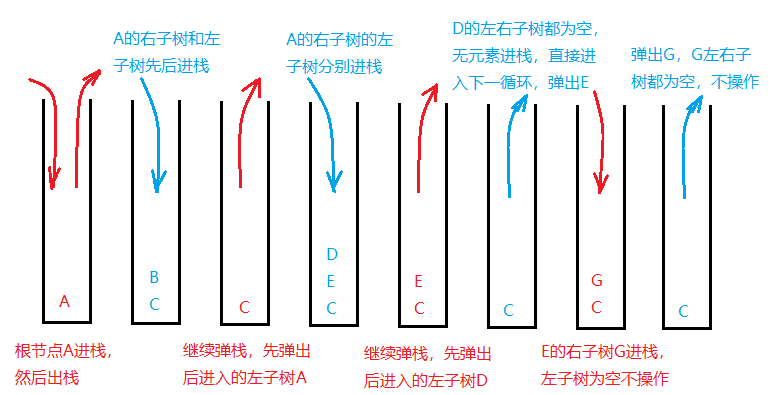public static void preNodeByStack(TreeNode node) {
if(node == null) {
return;
}

//创建一个栈，存放二叉树
Stack<TreeNode> stack = new Stack<>();
stack.push(node);

//将二叉树中的元素不断弹栈和压栈，直至二叉树为空
while(!stack.isEmpty()) {
//先弹出根节点
TreeNode n = stack.pop();
System.out.println(n.getValue());

//先将根节点的右孩子压栈，再将左孩子压榨，因为想先弹出左孩子，所以左孩子后进栈
if(n.rightChild != null) {
stack.push(n.rightChild);
}
if(n.leftChild != null) {
stack.push(n.leftChild);
}
}
}

1.直接创建结点，然后根据树的关系直接将每个结点左右子树的关系说明好

（1）创建A~J一共十个结点，并标好各自的下标

TreeNode nodeA = new TreeNode(1, "A");    ........

（2）将结点与自己的孩子进行联系

nodeA.leftChild = nodeB;

public static void createBinaryTree(TreeNode root) {
if(root ==null) {
return;
}
TreeNode nodeB = new TreeNode(2, "B");
TreeNode nodeC = new TreeNode(3, "C");
TreeNode nodeD = new TreeNode(4, "D");
TreeNode nodeE = new TreeNode(5, "E");
TreeNode nodeF = new TreeNode(6, "F");
TreeNode nodeG = new TreeNode(7, "G");
TreeNode nodeH = new TreeNode(8, "H");
TreeNode nodeI = new TreeNode(9, "I");
TreeNode nodeJ = new TreeNode(10, "J");

root.leftChild = nodeB;
nodeB.leftChild = nodeD;
nodeB.rightChild = nodeE;
nodeD.rightChild = nodeG;
root.rightChild = nodeC;
nodeC.rightChild = nodeF;
nodeF.leftChild = nodeH;
nodeF.rightChild = nodeI;
nodeI.rightChild = nodeJ;
}

2.将要创建的树构成一个完全二叉树，没有子树的时候用一个符号来代替，如#，下面红圈代表井号，使用前序遍历的方式创建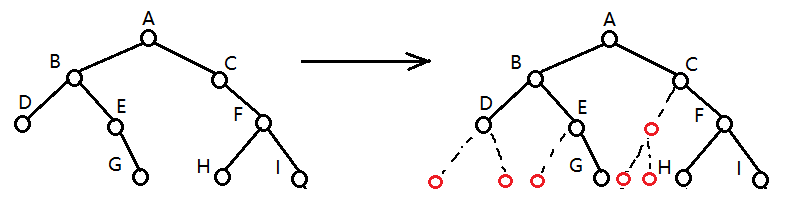//给定一个集合，用集合的元素按顺序创建树
public static void createBinaryTreePre(ArrayList<String> list) {
createBinaryTree2(list.size(), list);
}

public static TreeNode createBinaryTree2(int size, ArrayList<String> list) {
//集合为空时，返回null
if (list.size() == 0) {
return null;
}

//记录当前index，下标用于创建结点
int index = size - list.size();
TreeNode node;
//获取list中的第一个元素
String s = list.get(0);
//如果该元素为"#"，也就是说这个结点为空，那么移除当前元素，然后返回值为null的结点
if (s.equals("#")) {
node = null;
list.remove(0);
return node;
}

//如果结点不为空，那么创建一个新结点
node = new TreeNode(index, s);
//新结点的下标为0，说明是根结点
if (index == 0) {
root = node;
}
//无论是不是根结点，都要移除这个元素(目的是为了控制index的值)
list.remove(0);
//然后创建左结点和右结点，使用迭代的方式创建好整个树
node.leftChild = createBinaryTree2(size, list);
node.rightChild = createBinaryTree2(size, list);

return node;
}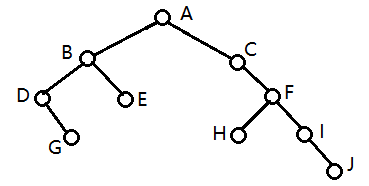1.建立二叉树的一个结点，每个结点有下标，元素值，左子树，右子树

2.计算二叉树的高度---二叉树的高度也就是将一条路走到黑的最长的距离，如上面的的树的的高度为5（A-C-F-I-J）

3.求二叉树的大小---也就是求二叉树一共有多少个结点

4.遍历二叉树

import java.util.Stack;

public class BinaryTree {

public static void main(String[] args) {
TreeNode root = new TreeNode(1, "A");
createBinaryTree(root);
System.out.println("Height : " + getHeight(root));
System.out.println("Size : " + getSize(root));
//        System.out.println("preNode : ");
//        preNode(root);
//        System.out.println("midNode : ");
//        midNode(root);
//        System.out.println("subNode :");
//        subNode(root);
System.out.println("preNodeByStack : ");
preNodeByStack(root);
}

//使用栈的方式遍历树---以前序遍历为例
public static void preNodeByStack(TreeNode node) {
if(node == null) {
return;
}

//创建一个栈，存放二叉树
Stack<TreeNode> stack = new Stack<>();
stack.push(node);

//将二叉树中的元素不断弹栈和压栈，直至二叉树为空
while(!stack.isEmpty()) {
//先弹出根节点
TreeNode n = stack.pop();
System.out.println(n.getValue());

//先将根节点的右孩子压栈，再将左孩子压榨，因为想先弹出左孩子，所以左孩子后进栈
if(n.rightChild != null) {
stack.push(n.rightChild);
}
if(n.leftChild != null) {
stack.push(n.leftChild);
}
}
}

//用后序遍历输出二叉树
public static void subNode(TreeNode node) {
if(node == null) {
return;
}

subNode(node.leftChild);
subNode(node.rightChild);
System.out.println(node.getValue());
}

//用中序遍历遍历二叉树并输出
public static void midNode(TreeNode node) {
if(node == null) {
return;
}

midNode(node.leftChild);
System.out.println(node.getValue());
midNode(node.rightChild);
}

//用前序遍历遍历二叉树并输出
public static void preNode(TreeNode node) {
if(node == null) {
return;
}
System.out.println(node.getValue());
preNode(node.leftChild);
preNode(node.rightChild);
}

//求二叉树的总结点大小，即二叉树的大小
public static int getSize(TreeNode node) {
if(node == null) {
return 0;
}
int i = getSize(node.leftChild);
int j = getSize(node.rightChild);
return 1 + i + j;
}

//求二叉树的高度
public static int getHeight(TreeNode node) {
if(node == null) {
return 0;
}
int i = getHeight(node.leftChild);
int j = getHeight(node.rightChild);
return i > j ? i + 1 : j + 1;
}

//创建一个二叉树
public static void createBinaryTree(TreeNode root) {
if(root ==null) {
return;
}
TreeNode nodeB = new TreeNode(2, "B");
TreeNode nodeC = new TreeNode(3, "C");
TreeNode nodeD = new TreeNode(4, "D");
TreeNode nodeE = new TreeNode(5, "E");
TreeNode nodeF = new TreeNode(6, "F");
TreeNode nodeG = new TreeNode(7, "G");
TreeNode nodeH = new TreeNode(8, "H");
TreeNode nodeI = new TreeNode(9, "I");
TreeNode nodeJ = new TreeNode(10, "J");

root.leftChild = nodeB;
nodeB.leftChild = nodeD;
nodeB.rightChild = nodeE;
nodeD.rightChild = nodeG;
root.rightChild = nodeC;
nodeC.rightChild = nodeF;
nodeF.leftChild = nodeH;
nodeF.rightChild = nodeI;
nodeI.rightChild = nodeJ;
}
}

//创建一个树

//结点类
class TreeNode {
private int index;
private String value;
//左右孩子初始值默认为null，因为建立这个点时，我们不知道其孩子的信息
TreeNode leftChild = null;
TreeNode rightChild = null;

public TreeNode(int index, String value) {
this.index = index;
this.value = value;
}

public int getIndex() {
return index;
}

public void setIndex(int index) {
this.index = index;
}

public String getValue() {
return value;
}

public void setValue(String value) {
this.value = value;
}
}

08-27985

12-071199
09-17174
08-192557
01-26363
03-171万+
05-311717
11-045万+
09-03858
12-19137
08-27863
02-0690
10-16914
11-10292
04-22211
10-265793
03-311409
06-243181
09-26348

### “相关推荐”对你有帮助么？

•非常没帮助
•没帮助
•一般
•有帮助
•非常有帮助szy2333

¥2 ¥4 ¥6 ¥10 ¥20余额支付 (余额：-- )扫码支付获取中扫码支付点击重新获取扫码支付1.余额是钱包充值的虚拟货币，按照1:1的比例进行支付金额的抵扣。
2.余额无法直接购买下载，可以购买VIP、C币套餐、付费专栏及课程。余额充值